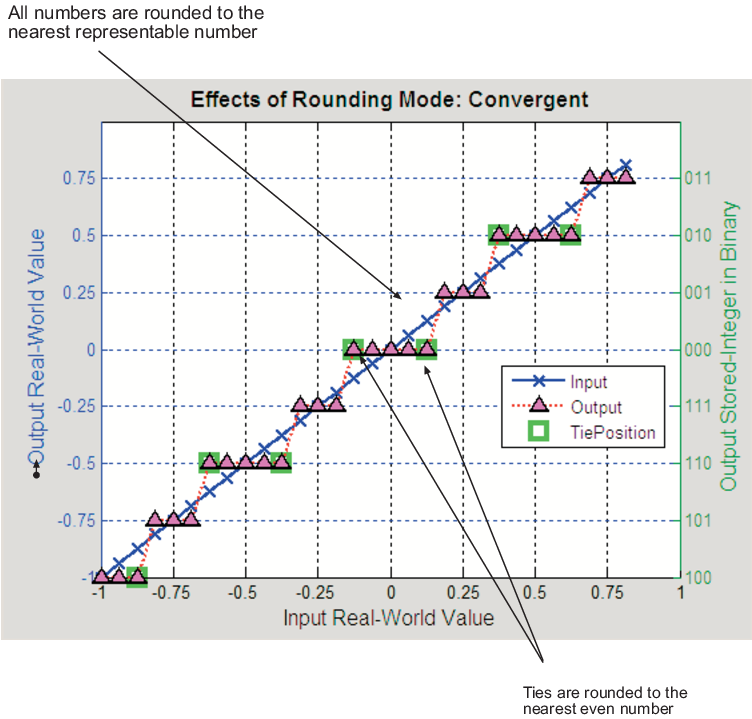## Rounding Mode: Convergent

`Convergent` rounds toward the nearest representable value with ties rounding toward the nearest even integer. It eliminates bias due to rounding. However, it introduces the possibility of overflow.

In the MATLAB® software, you can perform convergent rounding using the `convergent` function. `Convergent` rounding is shown in the following figure.## SupportGet trial now